# Can Acceleration Be Negative? (What It Means & 3 Key Ideas)

Acceleration is used in math and physics to describe how the velocity of an object changes.  However, it can be a little tricky to navigate the signs (positive and negative) of acceleration as it relates to velocity.

So, can acceleration be negative?  Acceleration can be negative when velocity is decreasing. Negative acceleration and positive velocity means decreasing speed (speed is moving towards zero). Negative acceleration and negative velocity means increasing speed. Deceleration occurs when acceleration and velocity have opposite signs.

Of course, negative acceleration is not theoretical, since it occurs in gravity and other natural phenomena.

In this article, we’ll talk about negative acceleration and when it can happen.  We’ll also look at some examples to make the concept clear.

Let’s get started.

## Can Acceleration Be Negative?

Acceleration can be negative, and this happens whenever velocity is decreasing.  This is because acceleration is the derivative of velocity.

That is, acceleration tells us how velocity is changing.  Negative acceleration means velocity is decreasing, and positive acceleration means velocity is increasing.

It is important to note that negative acceleration does not always mean a decrease in speed.  Since speed is the absolute value of velocity, we could have increasing or decreasing speed when acceleration is negative.

The table below helps to illustrate what happens with acceleration, velocity, and speed when they have different signs.

As the table suggests, negative velocity and negative acceleration means an object is speeding up: as the velocity becomes more negative, its absolute value (speed) increases.  We are really just speeding up in the negative direction.

Do not confuse deceleration with negative acceleration! Deceleration means a reduction in speed.

That is, the velocity moves towards zero, from either the positive or negative direction. An object moving forwards or backwards is coming closer to a stop (approaching zero velocity)

Another way to think of deceleration is “acceleration in a direction opposite to the direction of velocity.” This happens in the 3rd and 7th rows of the table above.

Note that for an object that is decelerating, the derivative of its speed function will be negative.

This also means that acceleration can be positive when an object is decelerating.  For example, if the object is traveling at -10 mph and slowing down to 0 mph, then acceleration is positive, but the object is decelerating as its speed approaches zero.

### What Does Negative Acceleration Mean?

Negative acceleration means decreasing velocity.  Negative acceleration indicates one of the following:

• The object is moving in a positive direction, and it is slowing down.  [positive velocity, decreasing speed]
• The object is at rest and is about to move backwards (in a negative direction).  [zero velocity, increasing speed]
• The object is moving in a negative direction, and it is speeding up.  [negative velocity, increasing speed]

As you can see, negative acceleration does not always indicate a decrease in speed.  If an object is at rest or moving in a negative direction, then a negative acceleration indicates an increase in speed.

### What Is The Difference Between Positive & Negative Acceleration?

The difference between positive and negative acceleration is the sign or direction of the change in velocity:

• Positive acceleration means that velocity is increasing (acceleration in the positive direction).
• Negative acceleration means that velocity is decreasing (acceleration in the negative direction).

### What Does Negative Acceleration Look Like On A Graph?

Negative acceleration looks like a concave (concave down) function on a graph of position.  This is because acceleration is the second derivative of position, and a negative second derivative indicates a concave function.

The graph could be a concave parabola, such as the one below, but there are other possibilities.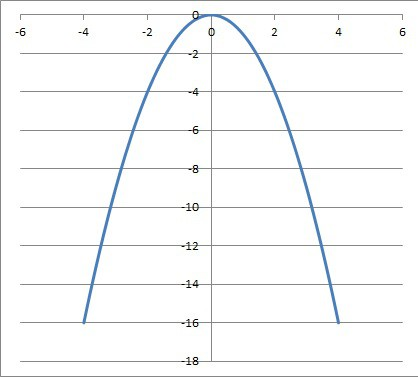A concave (or concave down) function has a negative second derivative. If this were a position function, then the acceleration would be negative (except at zero, where acceleration would be zero).

Negative acceleration looks like a decreasing (sloping down) function on a graph of velocity.  This is because acceleration is the first derivative of velocity, and a negative first derivative indicates a decreasing function.

The graph could be a line with a negative slope, such as the one below, but there are other possibilities.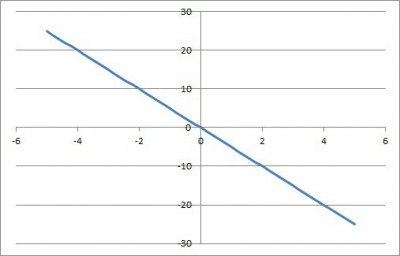A line with a negative slope moves down as we go right on the graph. If this were a position function, the velocity would have a constant negative value of -5.

### Can Acceleration Be Negative When Velocity Is Positive?

Acceleration can be negative when velocity is positive.  Remember that velocity is the first derivative of position, and acceleration is the second derivative of position (and the first derivative of velocity).

So, negative acceleration and positive velocity means that an object is moving in a positive direction (due to positive velocity), but it is slowing down (due to negative acceleration).

The graph of such a position function would be an increasing part of a concave curve, such as the one in the image below.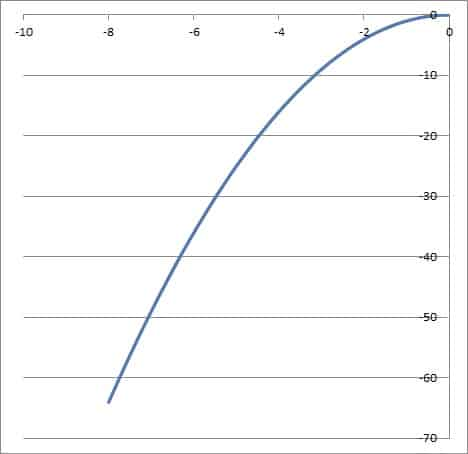This graph shows negative acceleration (concave down curve) and positive velocity (increasing curve). The object is moving in a positive direction, but at a slower and slower pace.

### Can Acceleration Be Negative When Velocity Is Zero?

Acceleration can be negative when velocity is zero.  Negative acceleration and zero velocity means that an object is standing still (due to zero velocity), but it is starting to move in a negative direction (due to negative acceleration).

The graph of such a position function would be a critical point (local maximum) of a concave curve, such as the vertex in the image below.This graph shows negative acceleration (concave down curve) and zero velocity (flat curve) at the vertex (time t = 0). The object is at rest at time t = 0, since its velocity is positive.

### Can Acceleration Be Negative When Velocity Is Negative?

Acceleration can be negative when velocity is negative.  Negative acceleration and negative velocity means that an object is moving in a negative direction (due to negative velocity), and it is speeding up (due to negative acceleration).

The graph of such a position function would be a decreasing part of a concave curve, such as the one in the image below.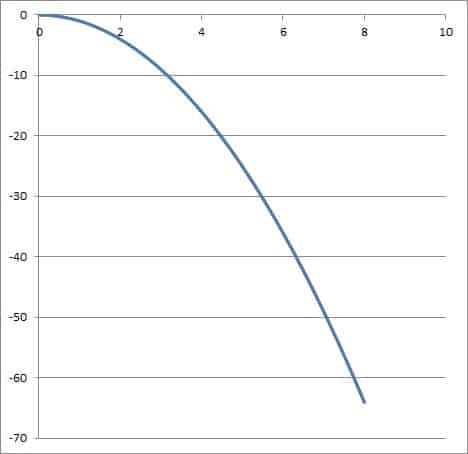This graph shows negative acceleration (concave down curve) and negative velocity (decreasing curve). The object is moving in a negative direction, and at a faster and faster pace.

## What Is An Example Of Negative Acceleration?

One common example of negative acceleration is the acceleration of an object due to the force of gravity on Earth.  The acceleration due to gravity is -9.8 m/s2 (or -32 ft/s2).

When dropped from rest, an object’s velocity will decrease by 9.8 m/s per second, due to the acceleration of gravity.

So, the object’s velocity would be:

• -9.8 meters per second after falling for 1 second
• 2*(-9.8) = -19.6 meters per second after falling for 2 seconds
• 3*(-9.8) = -29.4 meters per second after falling for 3 seconds
• … and so forth

You can see the graphs of position and velocity of an object dropped from rest in the graphs below.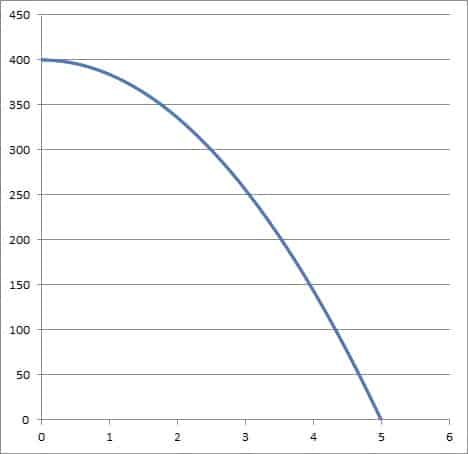This graph shows the position (height) graph of an object dropped from rest at a height of 400 feet and falling for 5 seconds. The acceleration due to gravity is -32 feet per second per second.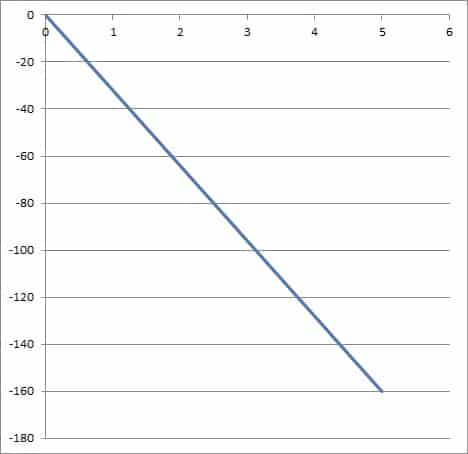This graph shows the velocity of an object dropped from rest at a height of 400 feet and falling for 5 seconds. The acceleration due to gravity is -32 feet per second per second. The velocity of the object when it hits the ground is -160 feet per second.

## Can Average Acceleration Be Negative?

Average acceleration can be negative over a time interval.  All that is required is that the final velocity is less than the starting velocity.

To prove this, remember that the formula for average acceleration over a time interval is:

• aavg = (vf – v0) / (tf – t0)

where t0 is the starting time, tf is the final time, v0 is the starting velocity (at time t0), and vf is the final velocity (at time tf).

Since the final time will be after the starting time, we know that the denominator tf – t0 will always be positive.

That means that the average acceleration will be negative if and only if the numerator is negative, or if:

• vf – v0 < 0
• vf < v0

That is, if the final velocity vf is less than the starting velocity v0.

### Example: When Average Acceleration Is Negative

Let’s say that a car has a starting velocity of 65 miles per hour at t = 1 hour.

At t = 4 hours, the car has a final velocity of 20 miles per hour.

To find the average acceleration over this time interval, we apply our formula from before:

• aavg = (vf – v0) / (tf – t0)
• aavg = (20 –65) / (4 – 1)
• aavg = (-45) / (3)
• aavg = -15

So, the average acceleration over this time interval is -15 miles per hour per hour.

That means that, on average, the car’s velocity decreased by 15 miles per hour for every hour we were driving.

Since there are 60 minutes in an hour, we could also say that on average, the car’s velocity decreased by 1 mile per hour every 4 minutes.

Of course, we don’t know how often the car changed speed between the starting and ending points.  It could have stopped completely, gone faster than 65 miles per hour, or both.

## Conclusion

Now you know about negative acceleration and how it happens.  You also know how negative acceleration relates to velocity and speed.